# Conditional Formatting for Rows with Similar Results

So you have a table neatly ordered by users, you proudly present it to your boss, waiting for the praise, but you forgot: She’s desperately near-sighted! How can she tell when the user_id changes without having to stress her eyes? If only there was a way to color coordinate rows based on when the user_id field changes..

Well with the below solutions, we can help get you the praise you deserve! Whether you’re using Python, Redshift on the Cache, or if you are querying your own Database, we have a fix for you.

Using Python

Periscope Data’s Python/R integration is especially handy for efficiently running loops over large data sets. Here, we compare the user_id from one record and compare it to the previous record to see if the value changed. If the value does change, we change the coloring of the user_id cell.

In your SQL editor, select the columns of the table you want to conditionally format. We’ve included a dataset from a fictional company below as an example. Be sure to order by the field you want to apply the conditional formatting to!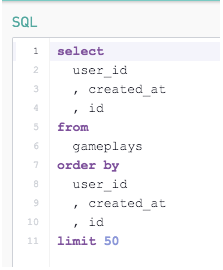Now, we use the following snippet. Notice how the snippet builds out a function that allows you to easily update the dataframe, column to conditionally format, and color choices

```# SQL output is imported as a pandas dataframe variable called "df"

import pandas as pd

import matplotlib.pyplot as plt

from pandas.tools.plotting import table

#Purpose:
#Inputs:
##dataframe (df) containing the table of data to be visualized
##column (col) to be conditionally formatted
##optional parameters to specify the hex code of the desired coloring (color1 and color2)
#Output: a matplotlib plot, plt

def color_block_formatting(df,col,color1="#e5c2fe",color2="#c2fefa"):
plt.figure(figsize=(10,15))
ax = plt.subplot(111, frame_on=False)
ax.xaxis.set_visible(False)
ax.yaxis.set_visible(False)

the_table=table(ax, df, rowLabels=['']*df.shape, bbox=[-0.1,0.1,1.2,1])
ax.axis('tight')

change=0
val=df[col]
for i in range(df.shape):
if val!=df[col][i]:
change+=1
if (change%2==0):
the_table._cells[(i+1, 0)].set_facecolor(color1)
else:
the_table._cells[(i+1, 0)].set_facecolor(color2)
val=df[col][i]

return plt

# Use Periscope to visualize a dataframe or an image by passing data to periscope.output()
periscope.output(color_block_formatting(df,col="user_id"))```

The command periscope.output(color_block_formatting(df,col="user_id")) returns the below Python Image. If your table is cut off, modify the following parameters:

• The figsize parameter of the plt.figure() call in line 14

• The bbox argument of the table function in line 19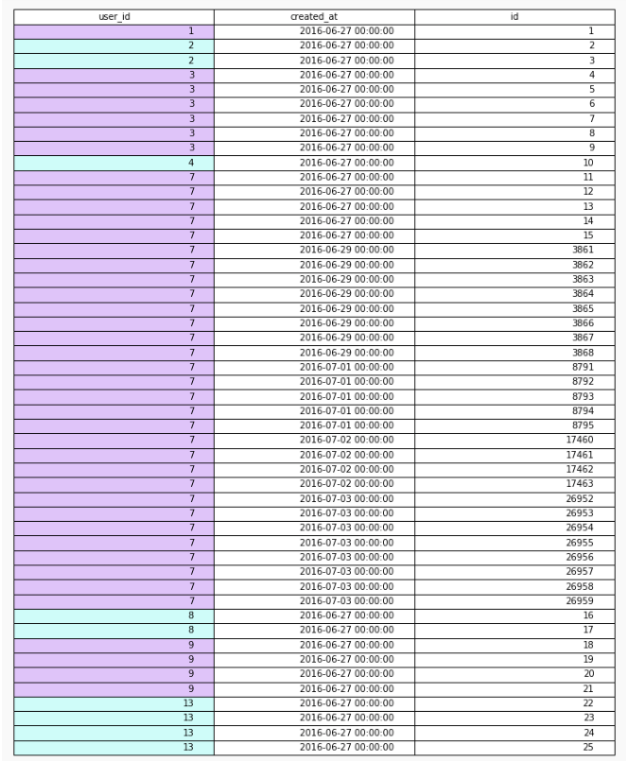Using the Cache (Redshift)

We’ll begin with the version of a gameplays table with proper ordering, but no conditional formatting.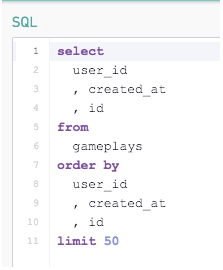What we need to do is add a column that will determine if the row has the same user_id as the row before it. With Redshift, you can get the prior row value using the LAG function: lag(user_id, 1)

Now we need to determine if this lagged value is the same as the current user_id. If it is, we should assign a value of ‘0’ to this new column. Else if it’s a new user_id, we should assign the column another value like ‘1’. We can do this by wrapping our LAG function in a CASE WHEN statement: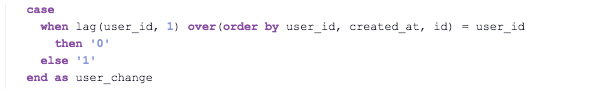Note: You want to use the over(order by) functions to make sure the lagged user_id is in the same order as your standard user_id!

Finally, we use the Conditional Formatting tool to assign a colors for the static values of the new column. Setting the same background and text color would avoid showing anything in the column all together, like this: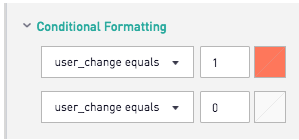So putting it all together, the code and table should look something like this: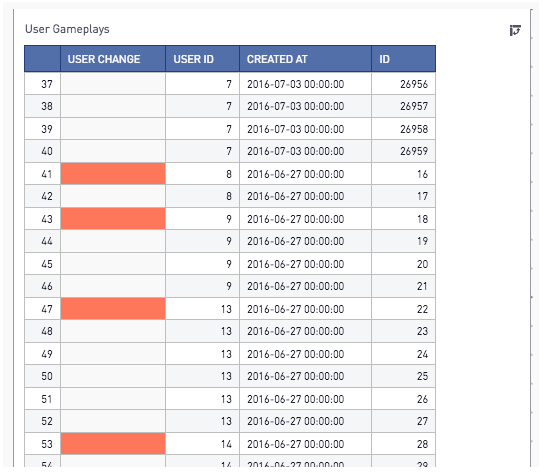Now you can quickly determine when the User_Id changes without having to look at the numbers themselves. Nicely done!

The above solution works great when you’re using the Cache or on your own Redshift database, but what if you didn’t have access to the LAG function? Well the workaround is still available, but requires a bit more SQL.

Another way to get the previous row’s value would be to join the table to a version of itself that is one row prior. To do this, your table must have a primary key. The syntax would look something like this: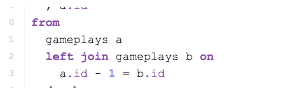Then you would create a CASE WHEN function to create a new column similar to the previous example: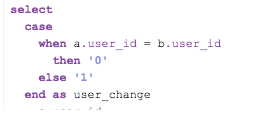Putting it all together, you should end up with the following: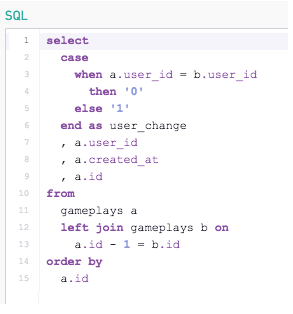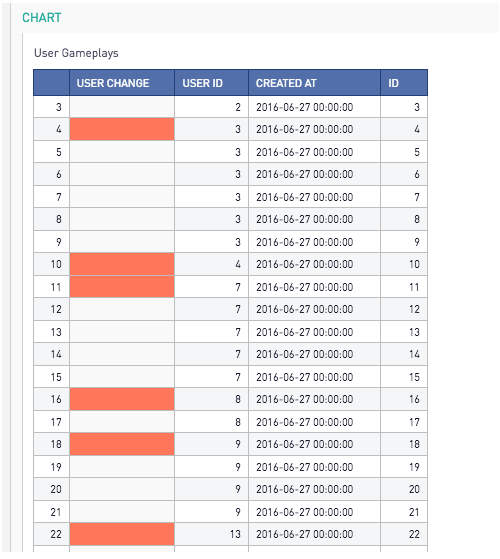Looking good!

Any of the above methods would help solve the complicated problem of dynamic formatting charts. Use your favorite, or use them all, to impress your boss or neighbor or family! Happy Periscoping!

2replies Oldest first
• Oldest first
• Popular•Same idea but using a Plotly table which can handle more rows and is easier to resize:

```import plotly
import plotly.graph_objs as go
import numpy as np
import pandas as pd
from datetime import datetime

colors = []
global val
val = df['id']
global change
change = 0
for i in range(df.shape):
if val != df['id'][i]:
change += 1
if (change % 2 == 0):
colors.append('#000000')
else:
colors.append('#DB3EB1')
val = df['issue_id'][i]

--the below is to make timestamps show on the table as timestamps instead of integers
df['timestamp'] = df['timestamp'].map(lambda t: t.strftime('%Y-%m-%d %H:%M'))
df['timestamp'] = (df['timestamp']).apply(str)

col = df.col.values
--and so on for all the columns^^^

trace = go.Table(columnwidth=[.06, .2, .2, .9, .3, .2, .2],
header=dict(values=['col2', 'col3', 'col4', 'col5', 'col6', 'col7'],
fill=dict(color='#41B6E6'),
font=dict(color='black', size=12),
align=['left']),
cells=dict(values=[col2, 'col3', 'col4', 'col5', 'col6', 'col7'],
fill=dict(color=[colors]),
align=['left'] * 8,
line=dict(color='#41B6E6'),
font=dict(color='white', size=12)))

data = [trace]

periscope.plotly(data)
```
•`from pandas.tools.plotting import table`
`from pandas.plotting import table`